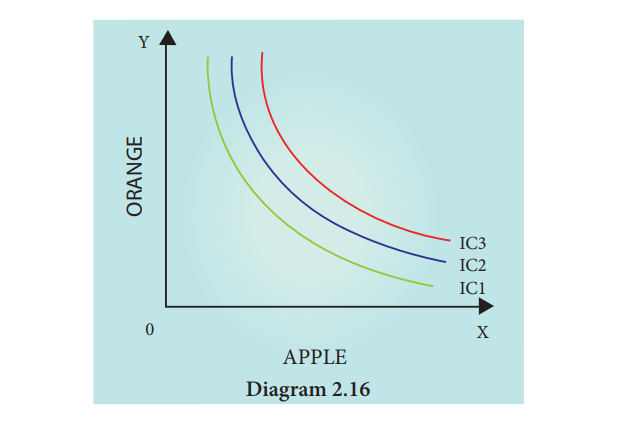Home | | Economics 11th std | An Indifference Map

# An Indifference MapOne can draw several indifference curves each representing an indifference schedule.

An Indifference Map

One can draw several indifference curves each representing an indifference schedule. Hence, an Indifference Map is a family or collection or set of indifference curves corresponding to different levels of satisfaction. The Indifference Map is illustrated in Diagram 2.16.In the diagram 2.16, the indifference Curves IC1, IC2 and IC3 represent the Indifference Map, Upper IC representing higher level of satisfaction compared to lower IC.

## Marginal Rate of Substitution

The shape of an indifference curve provides useful information about preferences. Indifference curve replaces the concept of marginal utility with the concept of the marginal rate of substitution.

According to Leftwich “The marginal rate of substitution of x for y (MRSxy) is defined as the maximum amount of y the consumer is willing to give up for getting an additional unit of x and still remaining on the same indifference curve”.

Tags : Economics , 11th Economics : Chapter 2 : Consumption Analysis
Study Material, Lecturing Notes, Assignment, Reference, Wiki description explanation, brief detail
11th Economics : Chapter 2 : Consumption Analysis : An Indifference Map | Economics